【欢迎转载，请注明作者：房燕良，原文出处：游戏程序员的自我修养

$L_o(p, v) = \int\limits_H (k_d\frac{c_{diff}}{\pi} + k_s\frac{D(h)F(l,h)G(l,v,h)}{4 (n \cdot l)(n \cdot v)})L_i(p,l) n \cdot l dl$

• 一部分的计算结果存储到一个 Cube Map 上，管它叫做“Pre-Filtered Environment Map”；
• 另外一部分的计算结果存储为一张 R16G16 格式的2D贴图，管它叫做：“Environment BRDF”。

【重要提示】 下面的内容需要两个背景知识：蒙特卡洛积分Image Based Lighting，如果你对这两不熟悉，可以先看文章后面一半的基础知识部分。

### Split Sum Approximation

$\int\limits_H L_i(p,l) f(l, v) cos \theta_l dl \approx \frac{1}{N} \sum_{k=1}^{N} \frac{L_i(l_k) f(l_k, v) cos \theta_{l_k}}{p(l_k, v)}$

$\frac{1}{N} \sum_{k=1}^{N} \frac{L_i(l_k) f(l_k, v) cos \theta_{l_k}}{p(l_k, v)} \approx (\frac{1}{N} \sum_{k=1}^{N} L_i(l_k) )(\frac{1}{N} \sum_{k=1}^{N} \frac{f(l_k, v) cos \theta_{l_k}}{p(l_k, v)} )$

#### 重要性采样（Importance Sampling）

float4 ImportanceSampleGGX( float2 E, float a2 )
{
float Phi = 2 * PI * E.x;
float CosTheta = sqrt( (1 - E.y) / ( 1 + (a2 - 1) * E.y ) );
float SinTheta = sqrt( 1 - CosTheta * CosTheta );

float3 H;
H.x = SinTheta * cos( Phi );
H.y = SinTheta * sin( Phi );
H.z = CosTheta;

float d = ( CosTheta * a2 - CosTheta ) * CosTheta + 1;
float D = a2 / ( PI*d*d );
float PDF = D * CosTheta;

return float4( H, PDF );
}


#### Hammersley Sequence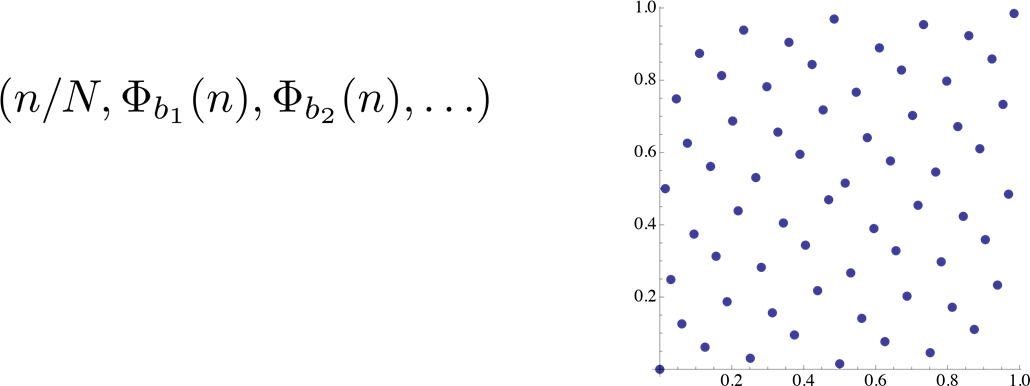float2 Hammersley( uint Index, uint NumSamples, uint2 Random )
{
float E1 = frac( (float)Index / NumSamples + float( Random.x & 0xffff ) / (1<<16) );
float E2 = float( ReverseBits32(Index) ^ Random.y ) * 2.3283064365386963e-10;
return float2( E1, E2 );
}


#### GGX Distribution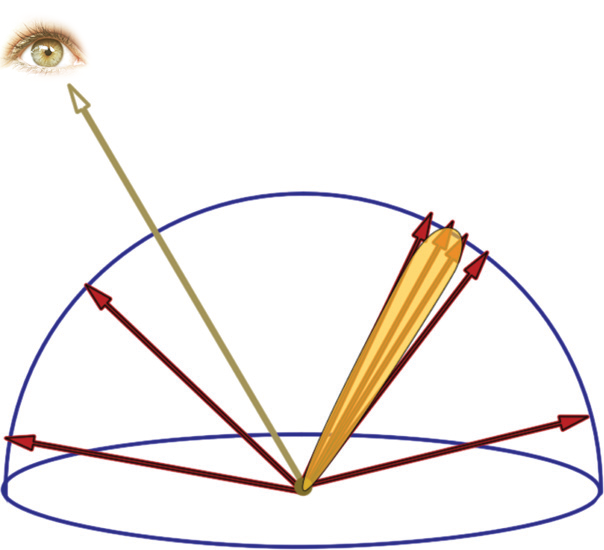### 计算Split Sum的第一部分

$\frac{1}{N} \sum_{k=1}^{N} L_i(l_k)$

1. 每一个 Mip Map Level 对应一个“粗糙度”值；
2. 对于每个 Mip Map Level，每一个贴图像素（texel）就对应一个反射方向；

float3 PrefilterEnvMap(float Roughness, float3 R)
{
float3 N = R;
float3 V = R;
float3 PrefilteredColor = 0;
const uint NumSamples = 1024;
for (uint i = 0; i < NumSamples; i++ )
{
float2 Xi = Hammersley(i, NumSamples);
float3 H = ImportanceSampleGGX(Xi, Roughness, N);
float3 L = 2 * dot(V, H) * H - V;
float NoL = saturate(dot(N, L));
if (NoL > 0) {
PrefilteredColor += EnvMap.SampleLevel(EnvMapSampler, L, 0).rgb * NoL;
TotalWeight += NoL;
}
}
return PrefilteredColor / TotalWeight;
}


### 计算Split Sum的第二部分

$\frac{1}{N} \sum_{k=1}^{N} \frac{f(l_k, v) cos \theta_{l_k}}{p(l_k, v)} = \int\limits_H f(l, v) cos \theta_l dl$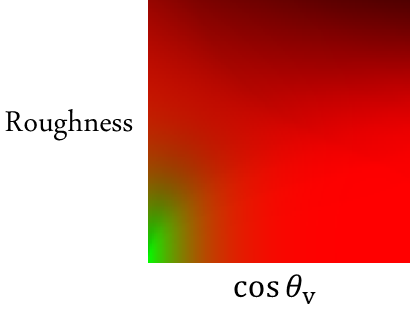$\int\limits_H f(l, v) cos \theta_l dl = F_0 \int\limits_H \frac {f(l, v)}{F(v,h)}(1 - (1 - v \cdot h)^5) cos \theta_l dl + \int\limits_H \frac {f(l, v)}{F(v,h)} (1 - v \cdot h)^5 cos \theta_l dl$

$\int\limits_H f(l, v) cos \theta_l dl = F_0 \cdot EnvBRDF.x + EnvBRDF.y$

float2 IntegrateBRDF(float Roughness, float NoV)
{
float3 V;
V.x = sqrt(1.0f - NoV * NoV); // sin
V.y = 0;
V.z = NoV; // cos
float A = 0;
float B = 0;
const uint NumSamples = 1024;
for (uint i = 0; i < NumSamples; i++ )
{
float2 Xi = Hammersley(i, NumSamples);
float3 H = ImportanceSampleGGX(Xi, Roughness, N);
float3 L = 2 * dot(V, H) * H - V;
float NoL = saturate(L.z);
float NoH = saturate(H.z);
float VoH = saturate(dot(V, H));
if (NoL > 0) {
float G = G_Smith(Roughness, NoV, NoL);
float G_Vis = G * VoH / (NoH * NoV);
float Fc = pow(1 - VoH, 5);
A += (1 - Fc) * G_Vis;
B += Fc * G_Vis;
}
}
return float2(A, B) / NumSamples;
}


### IBL 计算

float3 ApproximateSpecularIBL(float3 SpecularColor, float Roughness, float3 N, float3 V)
{
float NoV = saturate(dot(N, V));
float3 R = 2 * dot(V, N) * N - V;
float3 PrefilteredColor = PrefilterEnvMap(Roughness, R);
float2 EnvBRDF = IntegrateBRDF(Roughness, NoV);
return PrefilteredColor * (SpecularColor * EnvBRDF.x + EnvBRDF.y);
}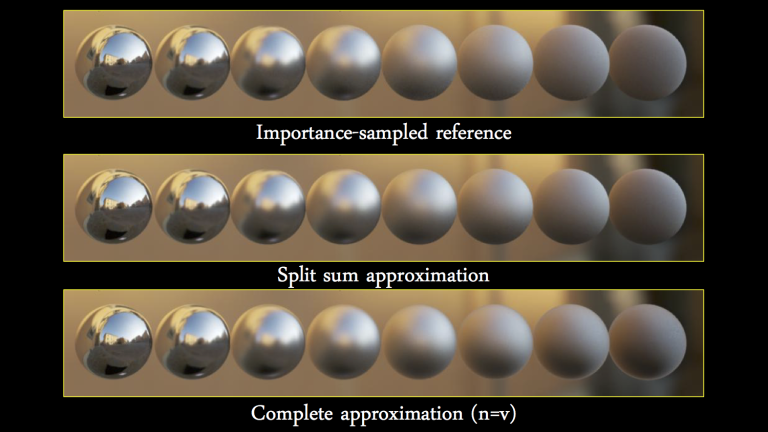### 基础知识

#### 蒙特卡洛积分（Monte Carlo integration）

$F = \int _{a}^{b}f(x)dx$

$F^{N} = \frac {1}{N}\sum _{i=1}^{N}\frac {f(X_{i})}{ pdf(X_{i}) }$

#### Image Based Lighting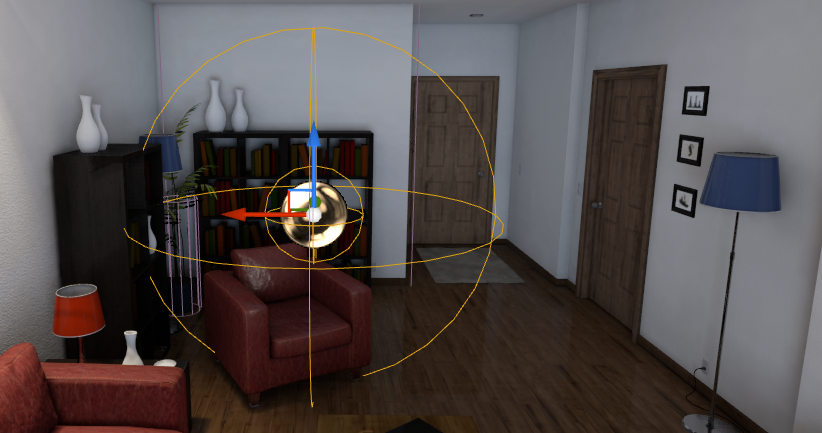1. 把场景中的静态环境，或者是天空，存储到一个环境贴图中；
2. 环境贴图中的每个像素可以想象成一个发射间接光的光源；使用 BRDF 对这些小小光源进行预计算，也就是所谓的卷积，从而生成 Light Probe；
3. 在运行时，根据需要着色的点的位置，找到合适的 Local Light Probes(可能是多个)，还有 Global Light Probe；
4. 分别根据粗糙度和反射向量，对这些 Light Probe 进行采样，然后插值；
5. 这个计算结果就是渲染中所需要的环境光反射量。